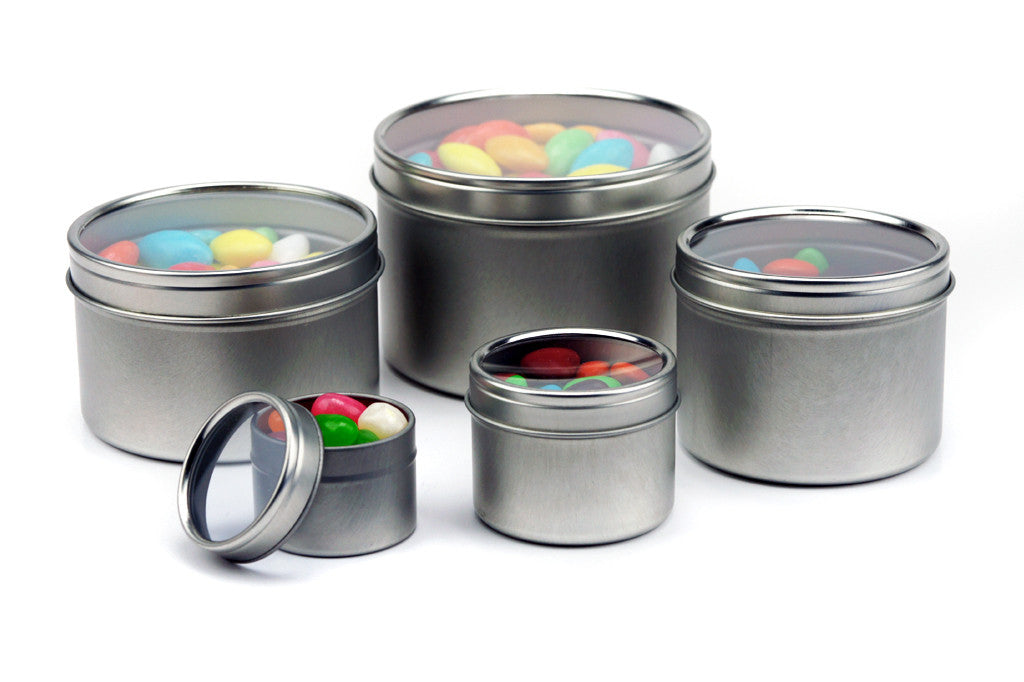Call Us : 626-988-4318

# Silver Round Deep Seamless Window Cover Slip Lid Metal Tin ContainersMimi Pack Silver Round Deep Seamless Window Cover Slip Lid Metal Tin Containers packaging consists of 24 tins. The tins have accurate tracks to guarantee a perfect fit on the twist tops and they are made of plated steel. The bottom and top part of the tins are flawlessly shaped and melded.

Volume(oz) Diameter(mm) Height(mm) Pack Quantity
1 39 28 24
2 50 37 24
4 62 44 24
6 69 52 24
8 80 50 24
10 95 50 24
14 95 64 24
16 102 64 24

Hit the "ADD TO CART" button and get yours today!
Size Color Item Number Dimensions
Diameter x Height(inches)
Quantity/Case Case Price(24) Unit Price Add To Cart
Black 1oz \$9.64
Liquid error: divided by 0 / unit
Blue 1oz \$9.64
Liquid error: divided by 0 / unit
Copper 1oz \$9.64
Liquid error: divided by 0 / unit
Gold 1oz \$9.64
Liquid error: divided by 0 / unit
Green 1oz \$9.64
Liquid error: divided by 0 / unit
Red 1oz \$9.64
Liquid error: divided by 0 / unit
Silver 1oz \$9.64
Liquid error: divided by 0 / unit
Black 2oz \$11.95
Liquid error: divided by 0 / unit
Blue 2oz \$11.95
Liquid error: divided by 0 / unit
Copper 2oz \$11.95
Liquid error: divided by 0 / unit
Gold 2oz \$11.95
Liquid error: divided by 0 / unit
Green 2oz \$11.95
Liquid error: divided by 0 / unit
Red 2oz \$11.95
Liquid error: divided by 0 / unit
Silver 2oz \$11.95
Liquid error: divided by 0 / unit
Black 4oz \$14.54
Liquid error: divided by 0 / unit
Blue 4oz \$14.54
Liquid error: divided by 0 / unit
Copper 4oz \$14.54
Liquid error: divided by 0 / unit
Gold 4oz \$14.54
Liquid error: divided by 0 / unit
Green 4oz \$14.54
Liquid error: divided by 0 / unit
Red 4oz \$14.54
Liquid error: divided by 0 / unit
Silver 4oz \$14.54
Liquid error: divided by 0 / unit
Black 6oz \$17.85
Liquid error: divided by 0 / unit
Blue 6oz \$17.85
Liquid error: divided by 0 / unit
Copper 6oz \$17.85
Liquid error: divided by 0 / unit
Gold 6oz \$17.85
Liquid error: divided by 0 / unit
Green 6oz \$17.85
Liquid error: divided by 0 / unit
Red 6oz \$17.85
Liquid error: divided by 0 / unit
Silver 6oz \$17.85
Liquid error: divided by 0 / unit
Black 8oz \$20.30
Liquid error: divided by 0 / unit
Blue 8oz \$20.30
Liquid error: divided by 0 / unit
Copper 8oz \$20.30
Liquid error: divided by 0 / unit
Gold 8oz \$20.30
Liquid error: divided by 0 / unit
Green 8oz \$20.30
Liquid error: divided by 0 / unit
Red 8oz \$20.30
Liquid error: divided by 0 / unit
Silver 8oz \$20.30
Liquid error: divided by 0 / unit
Black 10oz \$22.89
Liquid error: divided by 0 / unit
Blue 10oz \$22.89
Liquid error: divided by 0 / unit
Copper 10oz \$22.89
Liquid error: divided by 0 / unit
Gold 10oz \$22.89
Liquid error: divided by 0 / unit
Green 10oz \$22.89
Liquid error: divided by 0 / unit
Red 10oz \$22.89
Liquid error: divided by 0 / unit
Silver 10oz \$22.89
Liquid error: divided by 0 / unit
Black 14oz \$26.49
Liquid error: divided by 0 / unit
Blue 14oz \$26.49
Liquid error: divided by 0 / unit
Copper 14oz \$26.49
Liquid error: divided by 0 / unit
Gold 14oz \$26.49
Liquid error: divided by 0 / unit
Green 14oz \$26.49
Liquid error: divided by 0 / unit
Red 14oz \$26.49
Liquid error: divided by 0 / unit
Silver 14oz \$26.49
Liquid error: divided by 0 / unit
Black 16oz \$28.94
Liquid error: divided by 0 / unit
Blue 16oz \$28.94
Liquid error: divided by 0 / unit
Copper 16oz \$28.94
Liquid error: divided by 0 / unit
Gold 16oz \$28.94
Liquid error: divided by 0 / unit
Green 16oz \$28.94
Liquid error: divided by 0 / unit
Red 16oz \$28.94
Liquid error: divided by 0 / unit
Silver 16oz \$28.94
Liquid error: divided by 0 / unit